For the latest version of JMP Help, visit JMP.com/help.

Publication date: 11/29/2021

##Fit Statistics

The Fit Statistics report gives statistics used for model comparison. For all fit statistics, smaller is better. A likelihood ratio test between two models can be performed if one model is contained within the other. If not, a cautious comparison of likelihoods can be informative. For an example, see Fit a Spatial Structure Model.

Description of the Fit Statistics Report uses the following notation:

Specify the mixed model: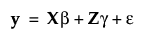Here y is the nx1 vector of observations, β is a vector of fixed-effect parameters, γ is a vector of random-effect parameters, and ε is a vector of errors.

The vectors γ and ε are assumed to have a multivariate normal distribution where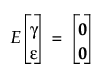and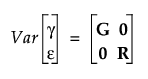With these assumptions, the variance of y is calculated as follows: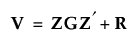#### Description of the Fit Statistics Report

-2 Residual Log Likelihood

The final evaluation of twice the negative residual log-likelihood, the objective function.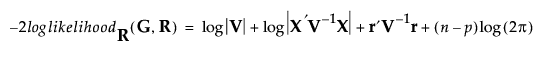where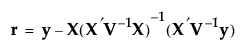and p is the rank of X. Use the residual likelihood only for model comparisons where the fixed effects portion of the model is identical. See Likelihood, AICc, and BIC.

-2 Log Likelihood

The evaluation of twice the negative log-likelihood function. See Likelihood, AICc, and BIC.

Use the log-likelihood for model comparisons in which the fixed, random, and repeated effects differ in any of the models.

AICc

Corrected Akaike’s Information Criterion. See Likelihood, AICc, and BIC.

BIC

Bayesian Information Criterion. See Likelihood, AICc, and BIC.

###Convergence Score Test

If there are problems with model convergence, a warning message is displayed below the fit statistics. Figure 8.11 shows the warning that suggests the cause and possible solutions to the convergence issue. It also includes a test of the relative gradient at the final iteration. If this test is non-significant, the model might be correct but not fully reaching the convergence criteria. In this case, consider using the model and results with caution. See Convergence Score Test.

Figure 8.11 Convergence Score Test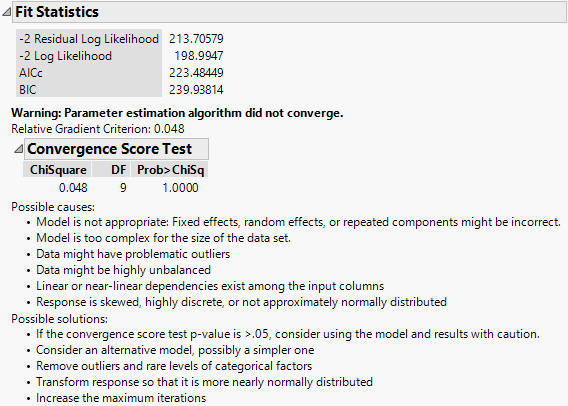Want more information? Have questions? Get answers in the JMP User Community (community.jmp.com).